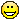1
|
15348 Views
|
3 Replies
|
3 Total Likes
View groups...
Share

# How to create 2D cross sections from 3D plot?

Posted 11 years ago
 Dear all,I have data (called "t20" here) of the form [{x1, y1, z1}, {x2, y2, z2}, ...}] which I have created a 3D plot with using ListPlot3D. I would really like to view cross sections along the xz and yz planes. After some fiddling around, I have:  Manipulate[  If[XorY == "y=c",    Grid[{{ListLinePlot[t20[[All, {1, 3}]] /. y -> c,        AxesLabel -> {"x", "z"}, PlotStyle -> Thick,        ImageSize -> {275, 275}],       ListPlot3D[t20, Mesh -> {{0}, {{c, Thick}}},        ImageSize -> {275, 275}]}}],    Grid[{{ListLinePlot[t20[[All, {2, 3}]] /. x -> c,        AxesLabel -> {"y", "z"}, PlotStyle -> Thick,       ImageSize -> {275, 275}],      ListPlot3D[t20, Mesh -> {{{c, Thick}}, {0}},       ImageSize -> {275, 275}]}}]], {{XorY, "x=c", "direction"}, {"x=c", "y=c"},   ControlType -> Setter}, {{c, 0, "c"}, 0, 500000,   Appearance -> "Labeled"}]which isn't quite right! How do i plot just an individual cross section rather than have them all stacked together?I guess the "All" in ListLinePlot[t20[[Allisn't right? Or have I done something else foolish?Please help! I'm sure this must be a simple problem to solve, but I'm not very familiar with using Mathematica. Suggestions welcome!!Many thanks,Smita.
3 Replies
Sort By:
Posted 11 years ago
 Aaaaahhhh THANK YOU!!! Kurt, you are awesomeThat is exactly what i needed! Thanks Sevy also, I'll have a look at the demonstration tooPosted 11 years ago
 i don't know if this helps, but a friend of mine uploaded this to the demonstrations project.  his code (downloadable from the right i believe) may help you.hope this helped, regards,SevyPosted 11 years ago
 You did not provide 't20' or a description of its structure, so I had to make my own.Hopefully it is compatible with yours:t20 = Table[   "inert"[{x, y, Sin[x Sin[y]]}], {x, 1, 10, .1}, {y, 1, 10, .1}];t20 = Cases[t20, "inert"[a___] :> a, Infinity];I think I achieved what you wanted with the following: Manipulate[  If[XorY == "y=c",    Grid[{{ListLinePlot[Cases[t20, {x_, c, z_} :> {x, z}],        AxesLabel -> {"x", "z"}, PlotStyle -> Thick,        ImageSize -> {275, 275}],       ListPlot3D[t20, Mesh -> {{0}, {{c, Thick}}},        ImageSize -> {275, 275}]}}],    Grid[{{ListLinePlot[Cases[t20, {c, y_, z_} :> {y, z}],        AxesLabel -> {"y", "z"}, PlotStyle -> Thick,       ImageSize -> {275, 275}],      ListPlot3D[t20, Mesh -> {{{c, Thick}}, {0}},       ImageSize -> {275, 275}]}}]], {{XorY, "x=c",    "direction"}, {"x=c", "y=c"},   ControlType -> Setter}, {{c, 1., "c"}, 1., 10., .1,   Appearance -> "Labeled"}]A matrix uses the Part[] or [[]] syntax.  However you do not seem to have a matrix.  You seem to have a list constaining lists of three coordinates.  That is, instead of having something such as {{1,2,3},{4,5,6}}, you have the equivalent information in a different form: {{1,1,1},{1,2,2},{1,3,3},{2,1,4},{2,2,5},{2,3,6}}.Cases[] would be a way to extract just the coordinates that you are interested in, such as the ones where the first coordinate (the 'x' coordinate) is equal to a constant 'c'.I would try to present this better but I am out of time for now.  I hope it helps you.